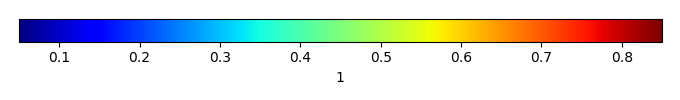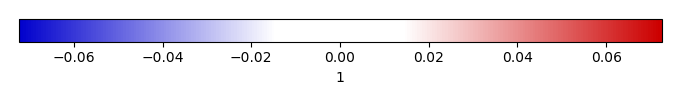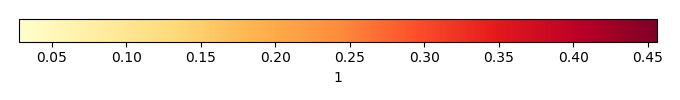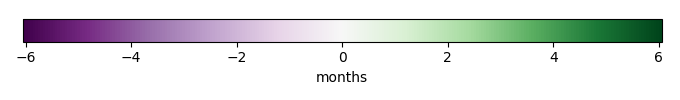# Mean State

Period Mean (original grids) 
Model Period Mean (intersection) 
Model Period Mean (complement) 
Benchmark Period Mean (intersection) 
Benchmark Period Mean (complement) 
Bias 
RMSE 
Phase Shift [months]
Bias Score 
RMSE Score 
Seasonal Cycle Score 
Spatial Distribution Score 
Interannual Variability Score 
Overall Score 
Benchmark [-] 0.175
CLM5PHSOFF [-] 0.158 0.157 0.00 0.181 0.0670 -0.0245 0.0514 3.84 0.28 0.33 0.33 0.99 0.48 0.45
CLM5PHSON [-] 0.155 0.154 0.00 0.181 0.0670 -0.0265 0.0522 3.97 0.26 0.33 0.31 0.99 0.47 0.45
Period Mean (original grids) 
Model Period Mean (intersection) 
Model Period Mean (complement) 
Benchmark Period Mean (intersection) 
Benchmark Period Mean (complement) 
Bias 
RMSE 
Phase Shift [months]
Bias Score 
RMSE Score 
Seasonal Cycle Score 
Spatial Distribution Score 
Interannual Variability Score 
Overall Score 
Benchmark [-] 0.215
CLM5PHSOFF [-] 0.190 0.192 0.00 0.217 0.0596 -0.0250 0.0553 4.20 0.36 0.29 0.27 0.99 0.50 0.45
CLM5PHSON [-] 0.186 0.188 0.00 0.217 0.0596 -0.0293 0.0559 3.74 0.33 0.31 0.36 0.99 0.49 0.46
Period Mean (original grids) 
Model Period Mean (intersection) 
Model Period Mean (complement) 
Benchmark Period Mean (intersection) 
Benchmark Period Mean (complement) 
Bias 
RMSE 
Phase Shift [months]
Bias Score 
RMSE Score 
Seasonal Cycle Score 
Spatial Distribution Score 
Interannual Variability Score 
Overall Score 
Benchmark [-] 0.168
CLM5PHSOFF [-] 0.158 0.158 0.00 0.173 0.0703 -0.0151 0.0525 4.21 0.30 0.27 0.26 0.90 0.56 0.43
CLM5PHSON [-] 0.157 0.157 0.00 0.173 0.0703 -0.0161 0.0509 3.99 0.29 0.29 0.30 0.88 0.53 0.43
Period Mean (original grids) 
Model Period Mean (intersection) 
Model Period Mean (complement) 
Benchmark Period Mean (intersection) 
Benchmark Period Mean (complement) 
Bias 
RMSE 
Phase Shift [months]
Bias Score 
RMSE Score 
Seasonal Cycle Score 
Spatial Distribution Score 
Interannual Variability Score 
Overall Score 
Benchmark [-] 0.281
CLM5PHSOFF [-] 0.355 0.355 0.00 0.281 0.273 0.0737 0.224 1.85 0.60 0.30 0.77 0.96 0.79 0.62
CLM5PHSON [-] 0.330 0.330 0.00 0.281 0.273 0.0483 0.189 1.54 0.69 0.34 0.83 0.96 0.78 0.66
Period Mean (original grids) 
Model Period Mean (intersection) 
Model Period Mean (complement) 
Benchmark Period Mean (intersection) 
Benchmark Period Mean (complement) 
Bias 
RMSE 
Phase Shift [months]
Bias Score 
RMSE Score 
Seasonal Cycle Score 
Spatial Distribution Score 
Interannual Variability Score 
Overall Score 
Benchmark [-] 0.113
CLM5PHSOFF [-] 0.138 0.138 0.00 0.126 0.0694 0.0115 0.0540 3.16 0.36 0.35 0.47 0.030 0.43 0.33
CLM5PHSON [-] 0.136 0.136 0.00 0.126 0.0694 0.00949 0.0536 3.13 0.34 0.36 0.48 0.018 0.40 0.33
Period Mean (original grids) 
Model Period Mean (intersection) 
Model Period Mean (complement) 
Benchmark Period Mean (intersection) 
Benchmark Period Mean (complement) 
Bias 
RMSE 
Phase Shift [months]
Bias Score 
RMSE Score 
Seasonal Cycle Score 
Spatial Distribution Score 
Interannual Variability Score 
Overall Score 
Benchmark [-] 0.170
CLM5PHSOFF [-] 0.235 0.234 0.00 0.170 0.125 0.0636 0.222 1.64 0.48 0.14 0.80 0.65 0.70 0.49
CLM5PHSON [-] 0.201 0.200 0.00 0.170 0.125 0.0295 0.145 1.53 0.64 0.23 0.82 0.73 0.70 0.56
Period Mean (original grids) 
Model Period Mean (intersection) 
Model Period Mean (complement) 
Benchmark Period Mean (intersection) 
Benchmark Period Mean (complement) 
Bias 
RMSE 
Phase Shift [months]
Bias Score 
RMSE Score 
Seasonal Cycle Score 
Spatial Distribution Score 
Interannual Variability Score 
Overall Score 
Benchmark [-] 0.176
CLM5PHSOFF [-] 0.206 0.206 0.00 0.179 0.0902 0.0274 0.0910 1.49 0.64 0.36 0.77 0.96 0.66 0.63
CLM5PHSON [-] 0.202 0.203 0.00 0.179 0.0902 0.0239 0.0841 1.49 0.65 0.38 0.78 0.96 0.65 0.63
Period Mean (original grids) 
Model Period Mean (intersection) 
Model Period Mean (complement) 
Benchmark Period Mean (intersection) 
Benchmark Period Mean (complement) 
Bias 
RMSE 
Phase Shift [months]
Bias Score 
RMSE Score 
Seasonal Cycle Score 
Spatial Distribution Score 
Interannual Variability Score 
Overall Score 
Benchmark [-] 0.142
CLM5PHSOFF [-] 0.160 0.160 0.00 0.148 0.0636 0.0116 0.0570 3.98 0.54 0.33 0.32 0.97 0.47 0.49
CLM5PHSON [-] 0.157 0.158 0.00 0.148 0.0636 0.00953 0.0556 3.96 0.54 0.34 0.31 0.97 0.44 0.49
Period Mean (original grids) 
Model Period Mean (intersection) 
Model Period Mean (complement) 
Benchmark Period Mean (intersection) 
Benchmark Period Mean (complement) 
Bias 
RMSE 
Phase Shift [months]
Bias Score 
RMSE Score 
Seasonal Cycle Score 
Spatial Distribution Score 
Interannual Variability Score 
Overall Score 
Benchmark [-] 0.307
CLM5PHSOFF [-] 0.356 0.353 0.00 0.306 0.330 0.0471 0.187 1.98 0.66 0.37 0.74 0.98 0.77 0.65
CLM5PHSON [-] 0.347 0.345 0.00 0.306 0.330 0.0384 0.172 1.85 0.70 0.39 0.77 0.98 0.76 0.66
Period Mean (original grids) 
Model Period Mean (intersection) 
Model Period Mean (complement) 
Benchmark Period Mean (intersection) 
Benchmark Period Mean (complement) 
Bias 
RMSE 
Phase Shift [months]
Bias Score 
RMSE Score 
Seasonal Cycle Score 
Spatial Distribution Score 
Interannual Variability Score 
Overall Score 
Benchmark [-] 0.152
CLM5PHSOFF [-] 0.171 0.171 0.00 0.159 0.0651 0.0125 0.0471 2.80 0.46 0.32 0.55 0.84 0.57 0.51
CLM5PHSON [-] 0.168 0.168 0.00 0.159 0.0651 0.00995 0.0467 2.64 0.44 0.32 0.58 0.82 0.56 0.51
Period Mean (original grids) 
Model Period Mean (intersection) 
Model Period Mean (complement) 
Benchmark Period Mean (intersection) 
Benchmark Period Mean (complement) 
Bias 
RMSE 
Phase Shift [months]
Bias Score 
RMSE Score 
Seasonal Cycle Score 
Spatial Distribution Score 
Interannual Variability Score 
Overall Score 
Benchmark [-] 0.146
CLM5PHSOFF [-] 0.170 0.170 0.00 0.154 0.0654 0.0169 0.0878 2.02 0.60 0.30 0.69 0.98 0.65 0.59
CLM5PHSON [-] 0.166 0.167 0.00 0.154 0.0654 0.0131 0.0829 1.98 0.60 0.32 0.70 0.97 0.66 0.59
Period Mean (original grids) 
Model Period Mean (intersection) 
Model Period Mean (complement) 
Benchmark Period Mean (intersection) 
Benchmark Period Mean (complement) 
Bias 
RMSE 
Phase Shift [months]
Bias Score 
RMSE Score 
Seasonal Cycle Score 
Spatial Distribution Score 
Interannual Variability Score 
Overall Score 
Benchmark [-] 0.135
CLM5PHSOFF [-] 0.275 0.275 0.00 0.240 0.0836 0.0356 0.100 2.44 0.48 0.33 0.61 0.98 0.57 0.55
CLM5PHSON [-] 0.269 0.269 0.00 0.240 0.0836 0.0298 0.0901 2.41 0.50 0.35 0.61 0.97 0.56 0.56
Period Mean (original grids) 
Model Period Mean (intersection) 
Model Period Mean (complement) 
Benchmark Period Mean (intersection) 
Benchmark Period Mean (complement) 
Bias 
RMSE 
Phase Shift [months]
Bias Score 
RMSE Score 
Seasonal Cycle Score 
Spatial Distribution Score 
Interannual Variability Score 
Overall Score 
Benchmark [-] 0.263
CLM5PHSOFF [-] 0.314 0.314 0.00 0.271 0.0684 0.0425 0.0749 1.57 0.35 0.36 0.80 0.99 0.52 0.56
CLM5PHSON [-] 0.313 0.313 0.00 0.271 0.0684 0.0416 0.0747 1.65 0.35 0.36 0.78 0.99 0.52 0.56
Period Mean (original grids) 
Model Period Mean (intersection) 
Model Period Mean (complement) 
Benchmark Period Mean (intersection) 
Benchmark Period Mean (complement) 
Bias 
RMSE 
Phase Shift [months]
Bias Score 
RMSE Score 
Seasonal Cycle Score 
Spatial Distribution Score 
Interannual Variability Score 
Overall Score 
Benchmark [-] 0.300
CLM5PHSOFF [-] 0.376 0.376 0.00 0.301 0.293 0.0751 0.216 1.85 0.61 0.35 0.76 0.96 0.78 0.63
CLM5PHSON [-] 0.366 0.366 0.00 0.301 0.293 0.0650 0.196 1.68 0.65 0.38 0.79 0.94 0.77 0.65
Period Mean (original grids) 
Model Period Mean (intersection) 
Model Period Mean (complement) 
Benchmark Period Mean (intersection) 
Benchmark Period Mean (complement) 
Bias 
RMSE 
Phase Shift [months]
Bias Score 
RMSE Score 
Seasonal Cycle Score 
Spatial Distribution Score 
Interannual Variability Score 
Overall Score 
Benchmark [-] 0.110
CLM5PHSOFF [-] 0.150 0.150 0.00 0.120 0.0597 0.0294 0.0509 3.35 0.44 0.32 0.44 0.79 0.53 0.47
CLM5PHSON [-] 0.148 0.148 0.00 0.120 0.0597 0.0275 0.0493 3.63 0.46 0.33 0.39 0.76 0.50 0.46
Period Mean (original grids) 
Model Period Mean (intersection) 
Model Period Mean (complement) 
Benchmark Period Mean (intersection) 
Benchmark Period Mean (complement) 
Bias 
RMSE 
Phase Shift [months]
Bias Score 
RMSE Score 
Seasonal Cycle Score 
Spatial Distribution Score 
Interannual Variability Score 
Overall Score 
Benchmark [-] 0.121
CLM5PHSOFF [-] 0.166 0.166 0.00 0.124 0.0591 0.0413 0.0840 2.26 0.48 0.33 0.64 0.90 0.64 0.55
CLM5PHSON [-] 0.159 0.159 0.00 0.124 0.0591 0.0348 0.0683 2.18 0.53 0.37 0.65 0.96 0.64 0.59
Period Mean (original grids) 
Model Period Mean (intersection) 
Model Period Mean (complement) 
Benchmark Period Mean (intersection) 
Benchmark Period Mean (complement) 
Bias 
RMSE 
Phase Shift [months]
Bias Score 
RMSE Score 
Seasonal Cycle Score 
Spatial Distribution Score 
Interannual Variability Score 
Overall Score 
Benchmark [-] 0.151
CLM5PHSOFF [-] 0.201 0.201 0.00 0.154 0.0803 0.0480 0.156 1.11 0.55 0.31 0.89 0.86 0.68 0.60
CLM5PHSON [-] 0.174 0.175 0.00 0.154 0.0803 0.0219 0.0997 1.05 0.67 0.42 0.89 0.93 0.65 0.67
Period Mean (original grids) 
Model Period Mean (intersection) 
Model Period Mean (complement) 
Benchmark Period Mean (intersection) 
Benchmark Period Mean (complement) 
Bias 
RMSE 
Phase Shift [months]
Bias Score 
RMSE Score 
Seasonal Cycle Score 
Spatial Distribution Score 
Interannual Variability Score 
Overall Score 
Benchmark [-] 0.159
CLM5PHSOFF [-] 0.185 0.185 0.00 0.161 0.0596 0.0237 0.0713 0.940 0.62 0.40 0.86 0.98 0.66 0.65
CLM5PHSON [-] 0.176 0.176 0.00 0.161 0.0596 0.0147 0.0589 1.10 0.67 0.44 0.83 0.99 0.68 0.67
Period Mean (original grids) 
Model Period Mean (intersection) 
Model Period Mean (complement) 
Benchmark Period Mean (intersection) 
Benchmark Period Mean (complement) 
Bias 
RMSE 
Phase Shift [months]
Bias Score 
RMSE Score 
Seasonal Cycle Score 
Spatial Distribution Score 
Interannual Variability Score 
Overall Score 
Benchmark [-] 0.133
CLM5PHSOFF [-] 0.137 0.137 0.00 0.135 0.0638 0.00176 0.0424 3.57 0.73 0.36 0.39 0.45 0.40 0.45
CLM5PHSON [-] 0.135 0.135 0.00 0.135 0.0638 -5.25e-05 0.0419 3.87 0.73 0.36 0.33 0.30 0.39 0.41
Period Mean (original grids) 
Model Period Mean (intersection) 
Model Period Mean (complement) 
Benchmark Period Mean (intersection) 
Benchmark Period Mean (complement) 
Bias 
RMSE 
Phase Shift [months]
Bias Score 
RMSE Score 
Seasonal Cycle Score 
Spatial Distribution Score 
Interannual Variability Score 
Overall Score 
Benchmark [-] 0.139
CLM5PHSOFF [-] 0.152 0.152 0.00 0.141 0.0683 0.0110 0.0462 4.58 0.65 0.31 0.19 0.91 0.48 0.48
CLM5PHSON [-] 0.149 0.149 0.00 0.141 0.0683 0.00773 0.0441 4.69 0.69 0.32 0.18 0.76 0.45 0.45
Period Mean (original grids) 
Model Period Mean (intersection) 
Model Period Mean (complement) 
Benchmark Period Mean (intersection) 
Benchmark Period Mean (complement) 
Bias 
RMSE 
Phase Shift [months]
Bias Score 
RMSE Score 
Seasonal Cycle Score 
Spatial Distribution Score 
Interannual Variability Score 
Overall Score 
Benchmark [-] 0.133
CLM5PHSOFF [-] 0.157 0.157 0.00 0.140 0.0680 0.0162 0.0660 3.25 0.45 0.30 0.46 0.90 0.56 0.50
CLM5PHSON [-] 0.159 0.159 0.00 0.140 0.0680 0.0185 0.0624 3.37 0.46 0.31 0.44 0.85 0.54 0.49

# Temporally integrated period mean

BENCHMARK MEANMODEL MEANBIASBIAS SCORERMSERMSE SCOREBENCHMARK INTERANNUAL VARIABILITYMODEL INTERANNUAL VARIABILITYINTERANNUAL VARIABILITY SCOREBENCHMARK MAX MONTHMODEL MAX MONTHDIFFERENCE IN MAX MONTHSEASONAL CYCLE SCORESPATIAL TAYLOR DIAGRAMMODEL COLORS# Spatially integrated regional mean

MODEL COLORSREGIONAL MEANANNUAL CYCLEMONTHLY ANOMALYANNUAL CYCLE# All Models

BenchmarkCLM5PHSOFFCLM5PHSON# Data Information

creation_date: Mon Jun 30 23:00:33 PDT 2014

source_file: This product is generated from monthly 1 degree GEWEX SRB Radiation observations